## Monday, July 02, 2012

### How do you determine if a number N is a Pentagonal Number?

Just yesterday, I decided to do a couple of problems from Project Euler. I hadn't been to the site in a long long time, but I was happy that I got through Problem 19 and Problem 43 in short order, but then I've temporarily hit a wall with Problem 44. The problem is phrased in terms of Pentagonal numbers and while working through it, I derived an interesting result that I'd like to share with you, namely "How do you efficiently test if a number is Pentagonal?".

Recall that a Pentagonal Number is a number N which is representable by a formula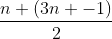where n is a Natural number. To test whether N is a Pentagonal Number, all we need to do is to solve the equation: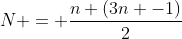which is a simple quadratic and check if n is a Natural Number. That's pretty easy for humans to do when N is a small quantity but programming languages aren't very good with quadratic equations, so we need to do some pre-processing of the quadratic equation for them.

Simplifying the above equation, we get: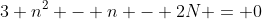The roots of this are: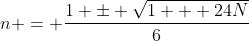Let's analyze the first root: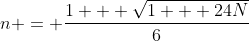Now, since n is a Natural number, 1 + sqrt(1 + 24N) should be divisible by 6. In more mathematical notation,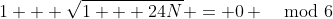(Note: Here I'm using the mathematical notation of mod rather than the CS notation of mod. The mod 6 at the end applies to both sides of the equation.)

Simple analysis would then indicate that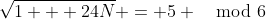Just checking the above condition is enough to determine if a number N is a non-generalized Pentagonal Number. But can we do better?

It is well known that the following property holds in modular arithmetic: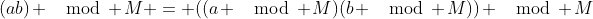Applying the above property to square the previous equation results in: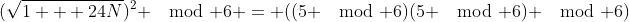or,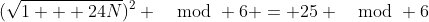or,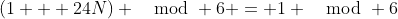or,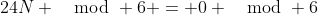The above constraint is always true, irrespective of the value of N. Therefore, the only constraints on testing if a number is Pentagonal is that 1 + 24 N must be a perfect square. A small caveat though is that we've used a squaring procedure to map x mod 6 to x^2 mod 6 - this mapping is not 1:1 since a squared number may have multiple roots in mod 6 space (indeed 1 mod 6 has two discrete square roots 1 mod 6 and 5 mod 6). What that means is that this test is actually necessary but not sufficient.

For numbers where (1 + 24 N) is a perfect square but sqrt(1 + 24 N) == 1 mod 6, the above squared equations are satisfied but they are not "normal" Pentagonal numbers. A quick glance will show that numbers which have sqrt(1 + 24 N) == 1 mod 6, when put into the formula for the root of the quadratic equation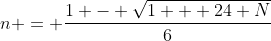(note the negative sign) will always yield a value that is 0 mod 6 thus guaranteeing an integral value for n (though negative in sign).

In other words, either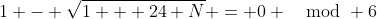or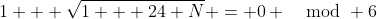always holds if (1 + 24 N) is a perfect square. Thus, we will always get an integral n (though not necessarily positive) as long as 1 + 24 N is a perfect square.

Therefore, in all cases, to test if a number is a Generalized Pentagonal Number, it is necessary and sufficient that (1 + 24 N) is a perfect square. If you want to stick to just non-Generalized Pentagonal numbers, then use the additional restriction that sqrt(1 + 24 N) mod 6 == 5. QED.

NOTE: A previous version of this post claimed the simpler test to be necessary and sufficient for non-Generalized Pentagonal Numbers. There was a flaw in the analysis and the post has been updated to indicate that the simpler test holds only for Generalized Pentagonal Numbers. Many thanks to Dave Evans for providing a detailed counterexample over email and apologies for the incorrect analysis and claim the first time around.

1.2.